# Overfitting example using hurricane frequencies

## 2021-12-11

### Overfitting

Overfitting occurs when a statistical model is too complex for the amount of data that it is based on. The summary statistics on the data itself appear to be quite good, but the model will almost always produce poor predictions for new data. Here is an example of overfitting using data on hurricane frequencies from the Data and Story Library site.

### The data used in this example (1/2)

##      Decade Count1851.1860 X6
## 1 1861-1870              1 NA
## 2 1871-1880              7 NA
## 3 1881-1890              5 NA
## 4 1891-1900              8 NA
## 5 1901-1910              4 NA
## 6 1911-1920              7 NA
## 7 1921-1930              5 NA
## 8 1931-1940              8 NA

Here are the first eight rows of data.

### The data used in this example (2/2)

##       Decade Count1851.1860 X6
## 9  1941-1950             10 NA
## 10 1951-1960              9 NA
## 11 1961-1970              6 NA
## 12 1971-1980              4 NA
## 13 1981-1990              4 NA
## 14 1991-2000              5 NA
## 15 2001-2006              7 NA
## NA      <NA>             NA NA

Here is the rest of the data.

### Plot the data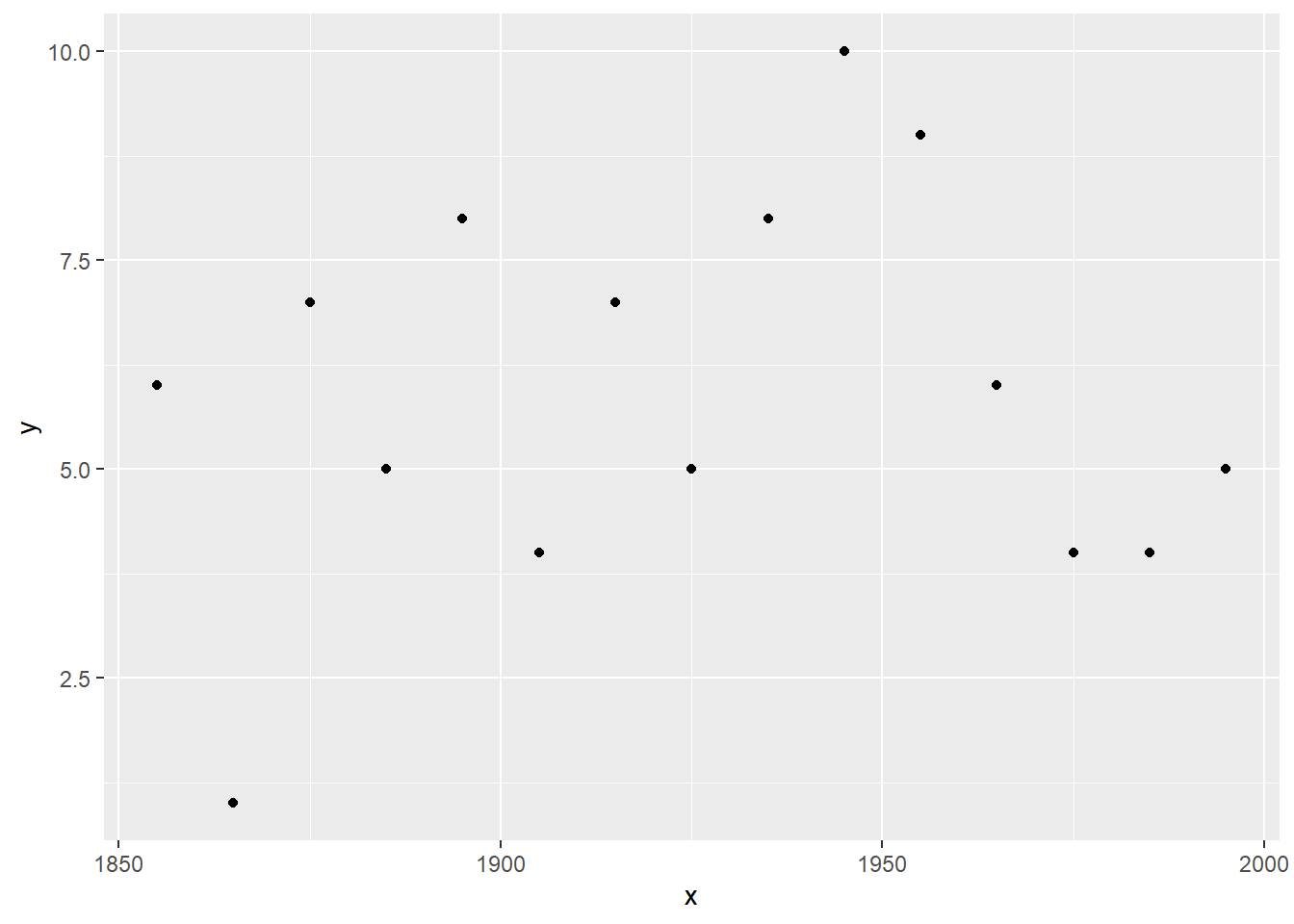Here’s what the data looks like in a graph. Nothing too unusual about the data. Now let’s try to forecast the number of hurricanes for the next decade.

### Plot the data with a linear prediction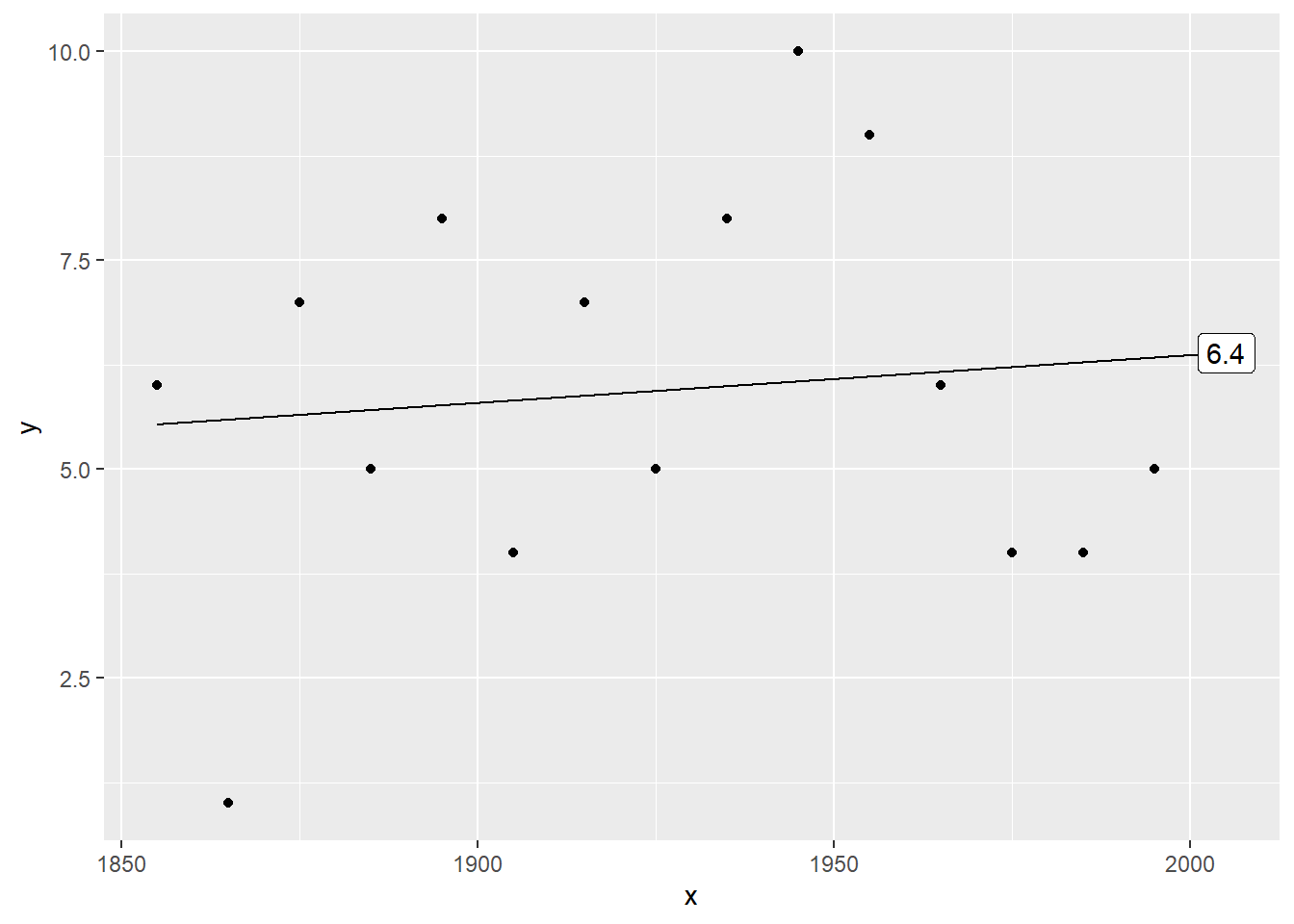Here’s a linear trend. The prediction is 6.6857143 for the next decade.

### Plot the data with a quadratic prediction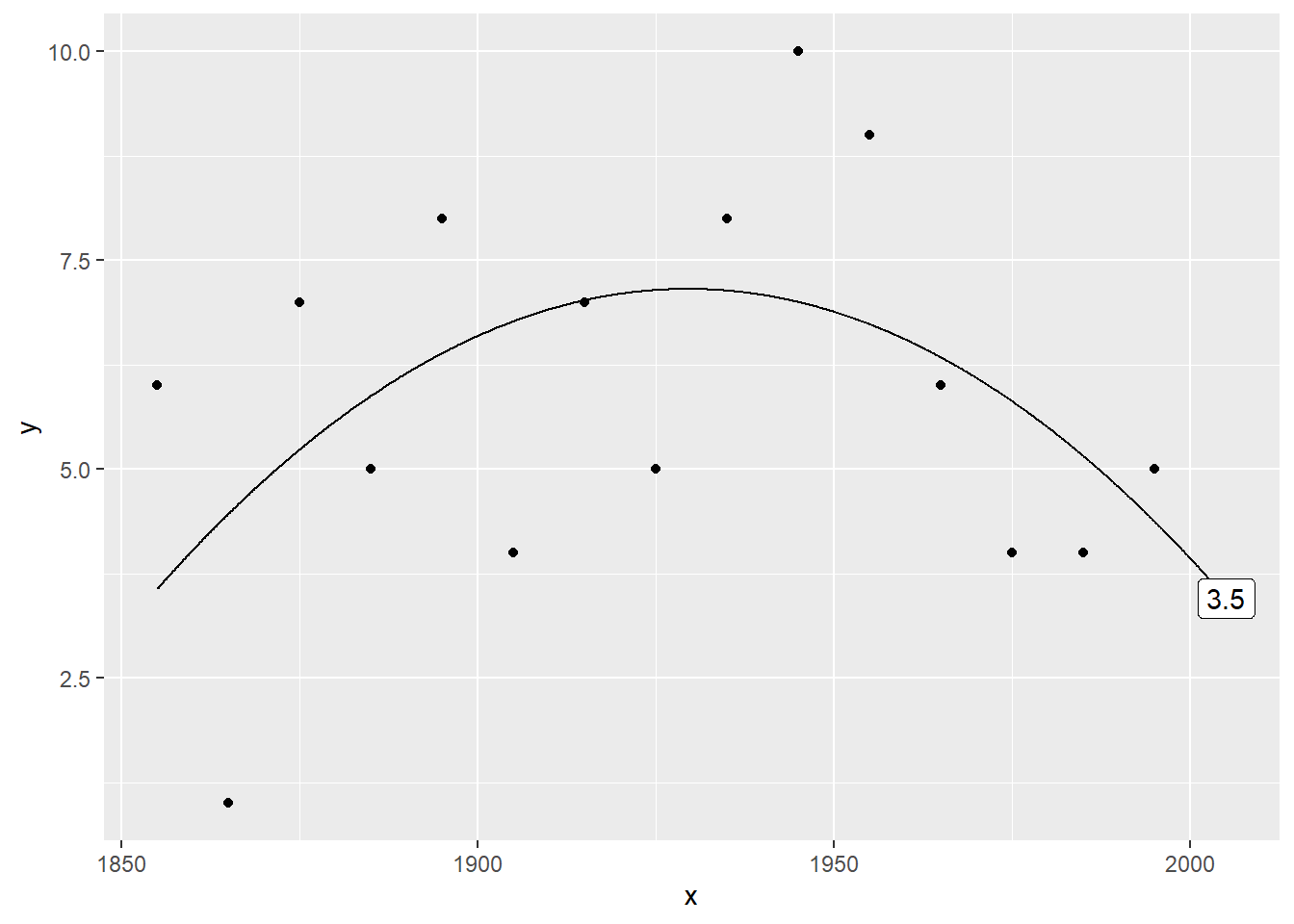Here’s a quadratic trend. The prediction is 3.7186813. It’s quite a bit different.

### Plot the data with a cubic prediction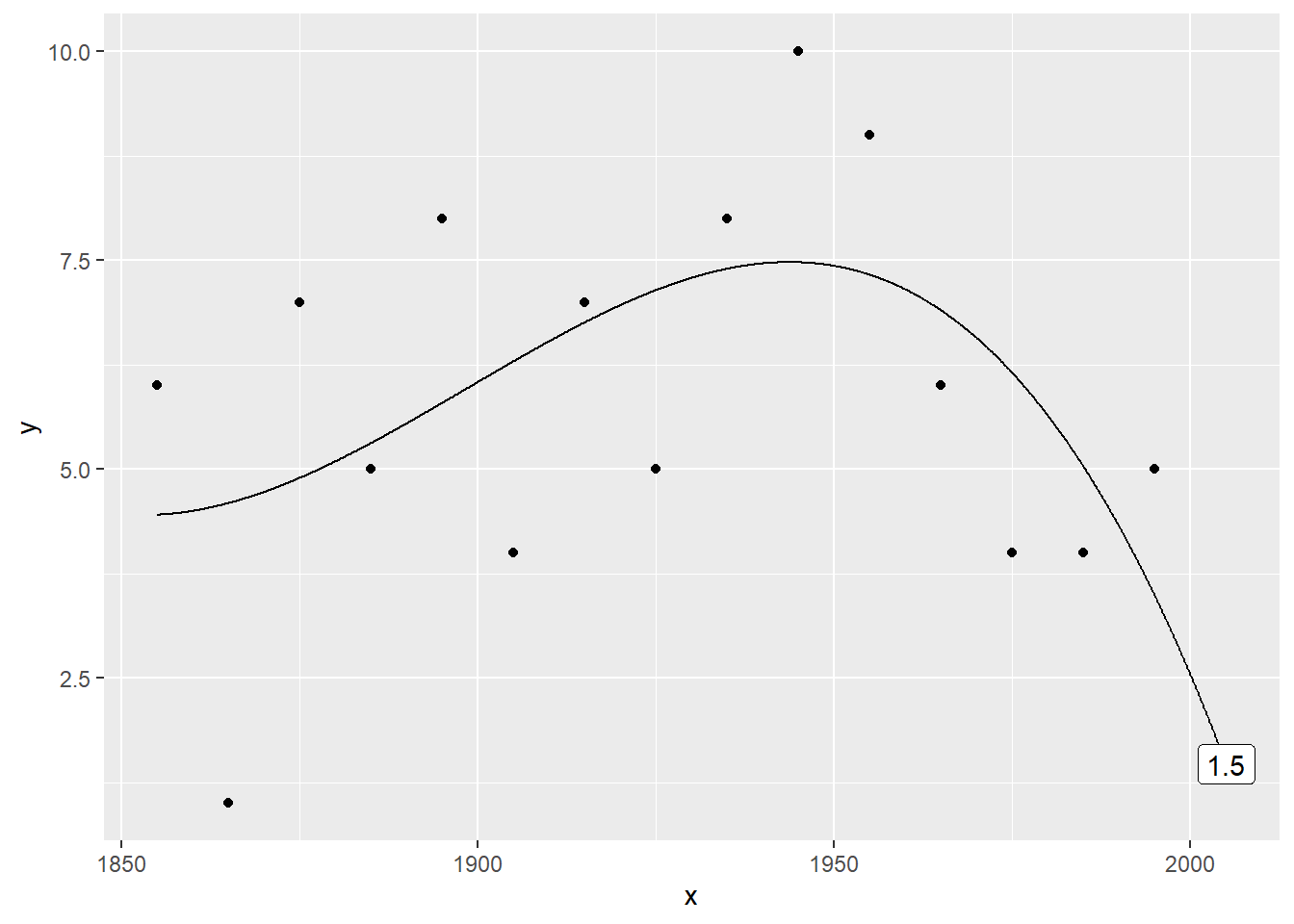Here’s a cubic (third order polynomial trend. The prediction is now 5.9340659.

### Plot the data with a 4th order polynomial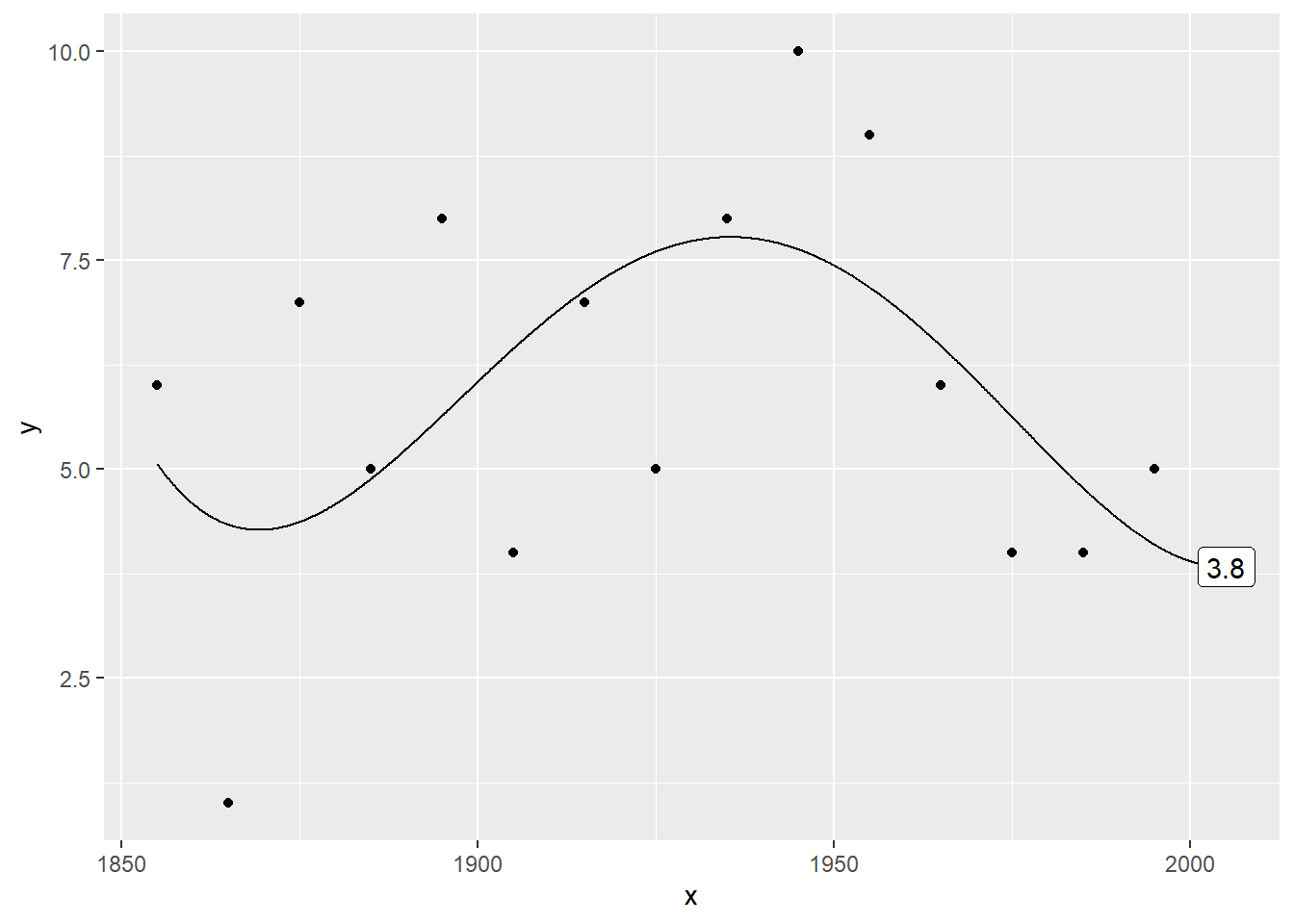Here’s a quartic (fourth order polynomial) trend. The prediction is 8.01998.

### Plot the data with a 5th order polynomial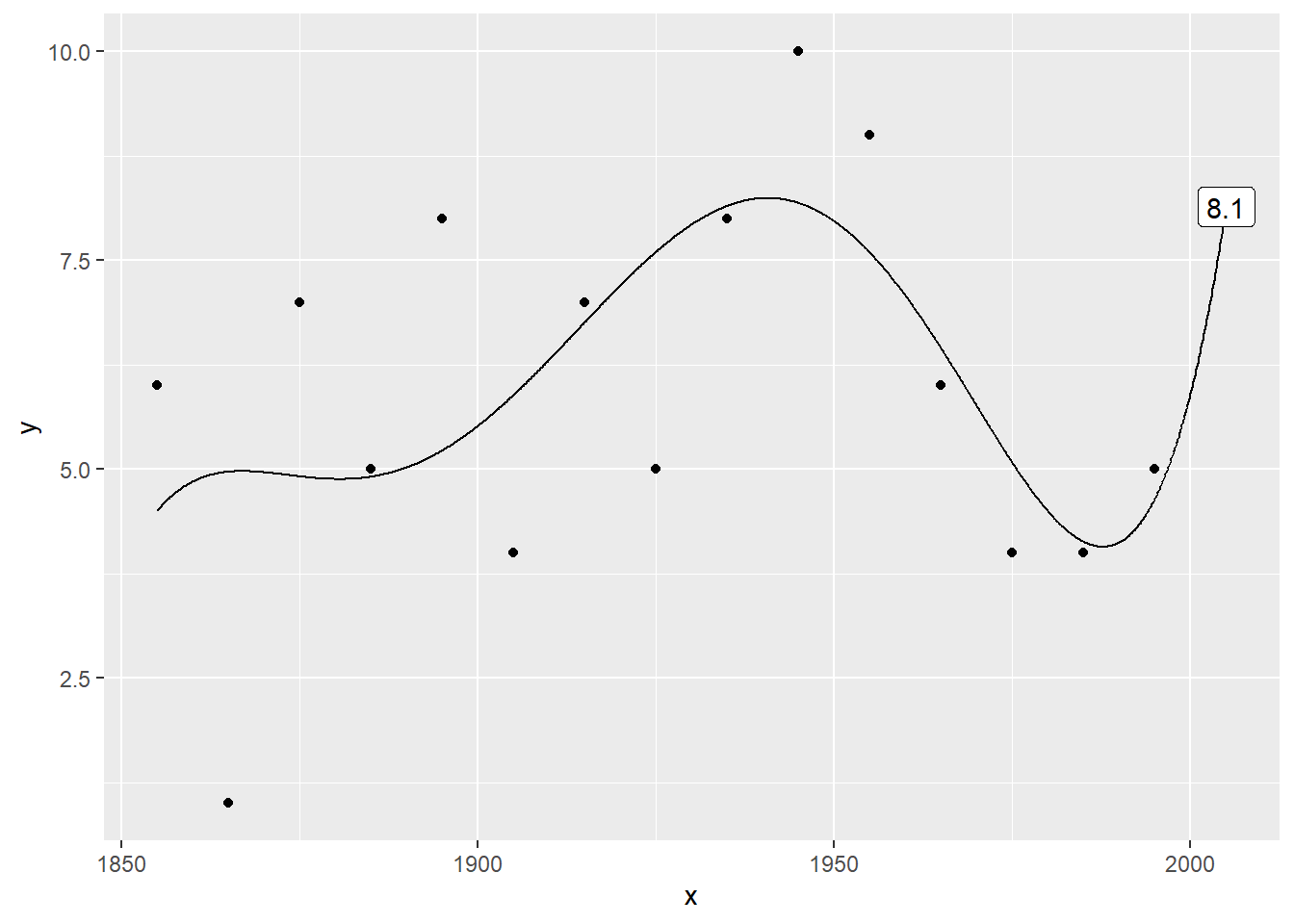Here’s a fifth order polynomial trend. The prediction is 19.9804196.

### Plot the data with a 6th order polynomial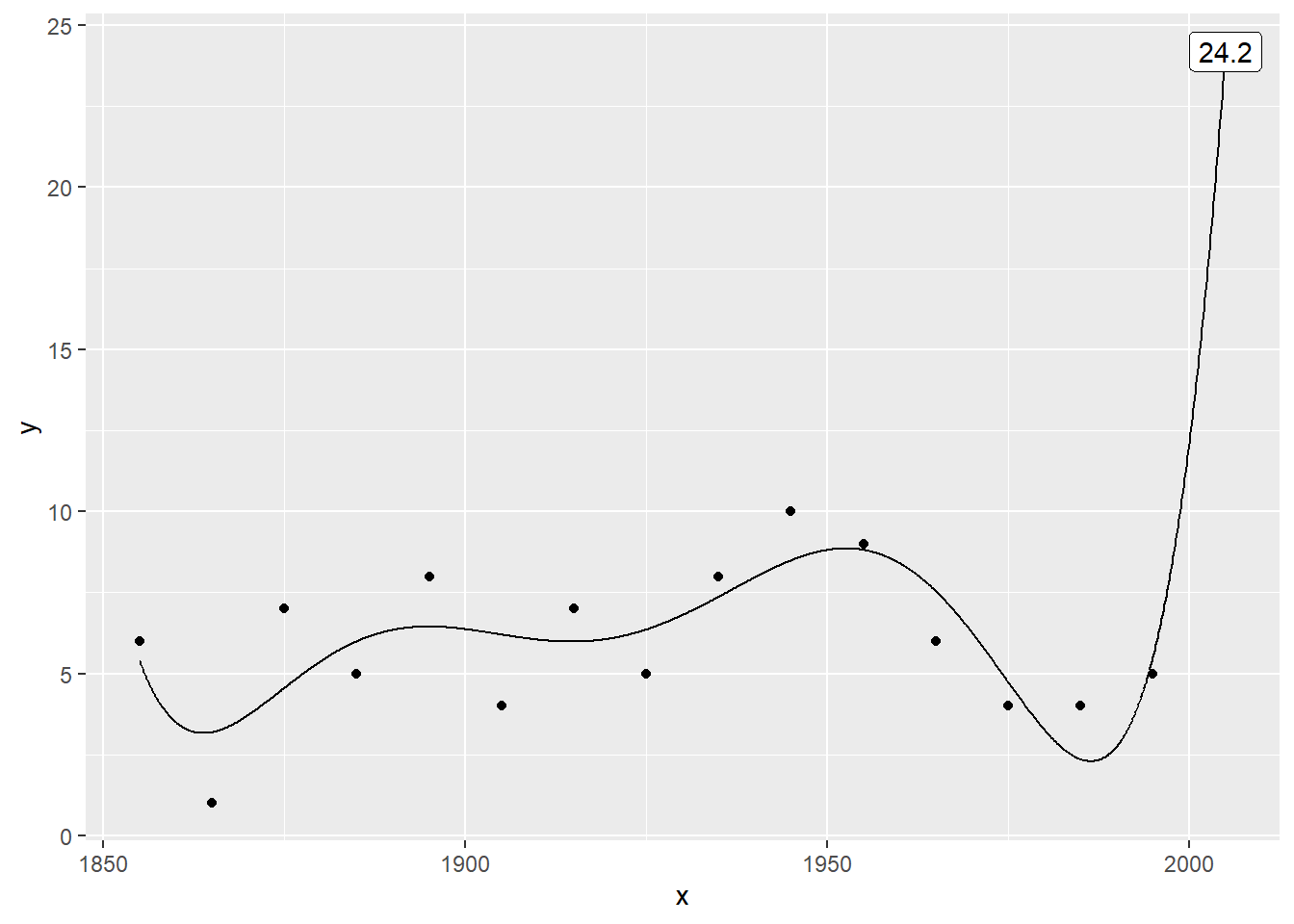Here’s where things get very weird. The sixth order polynomial produces a prediction of 11.6410256, which is more than twice as large as any previous value.

### Plot the data with a 7th order polynomial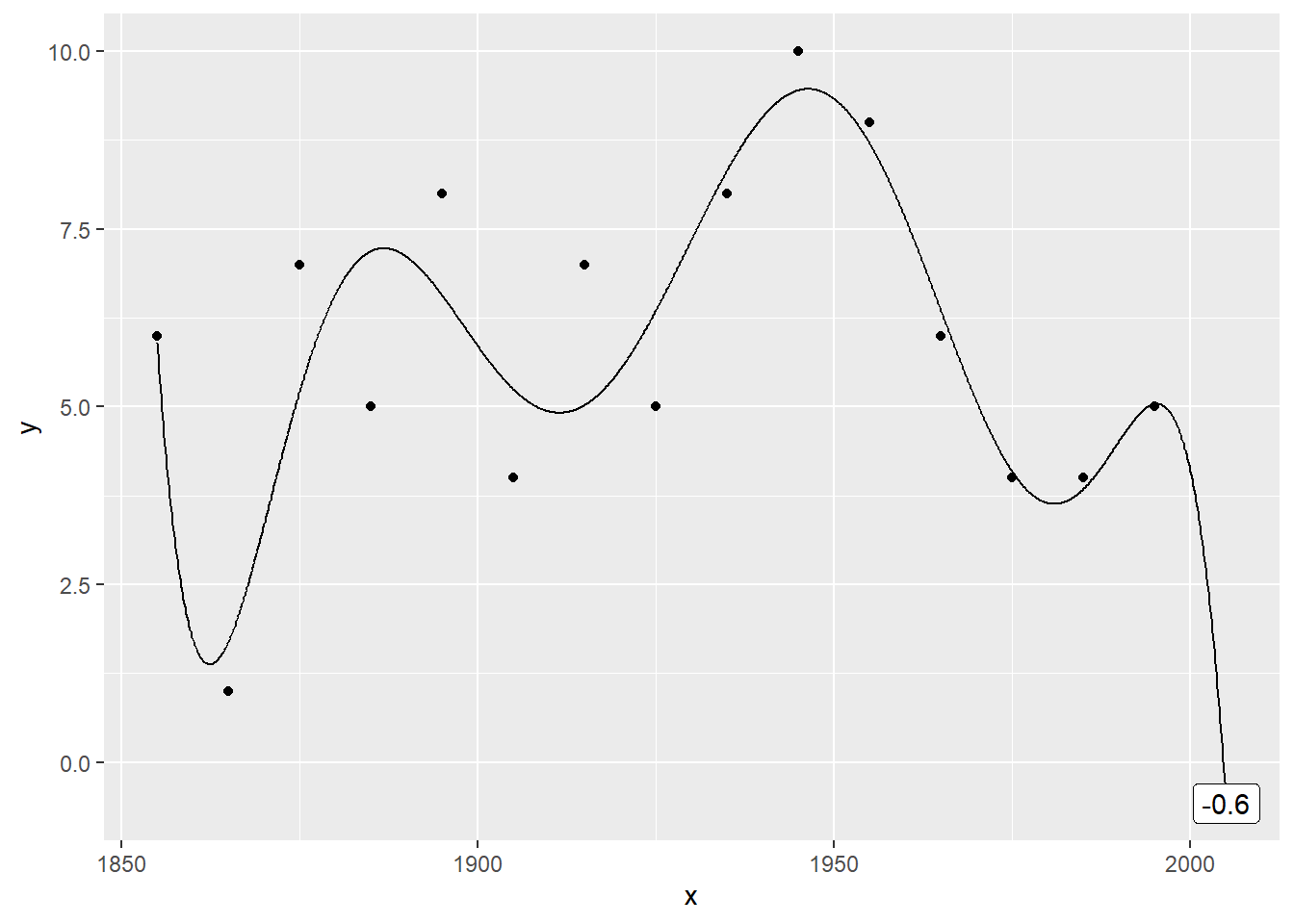The weirdness continues with the seventh order polynomial trend, which produces a negative prediction (-0.2051282.

### Plot the data with a 8th order polynomial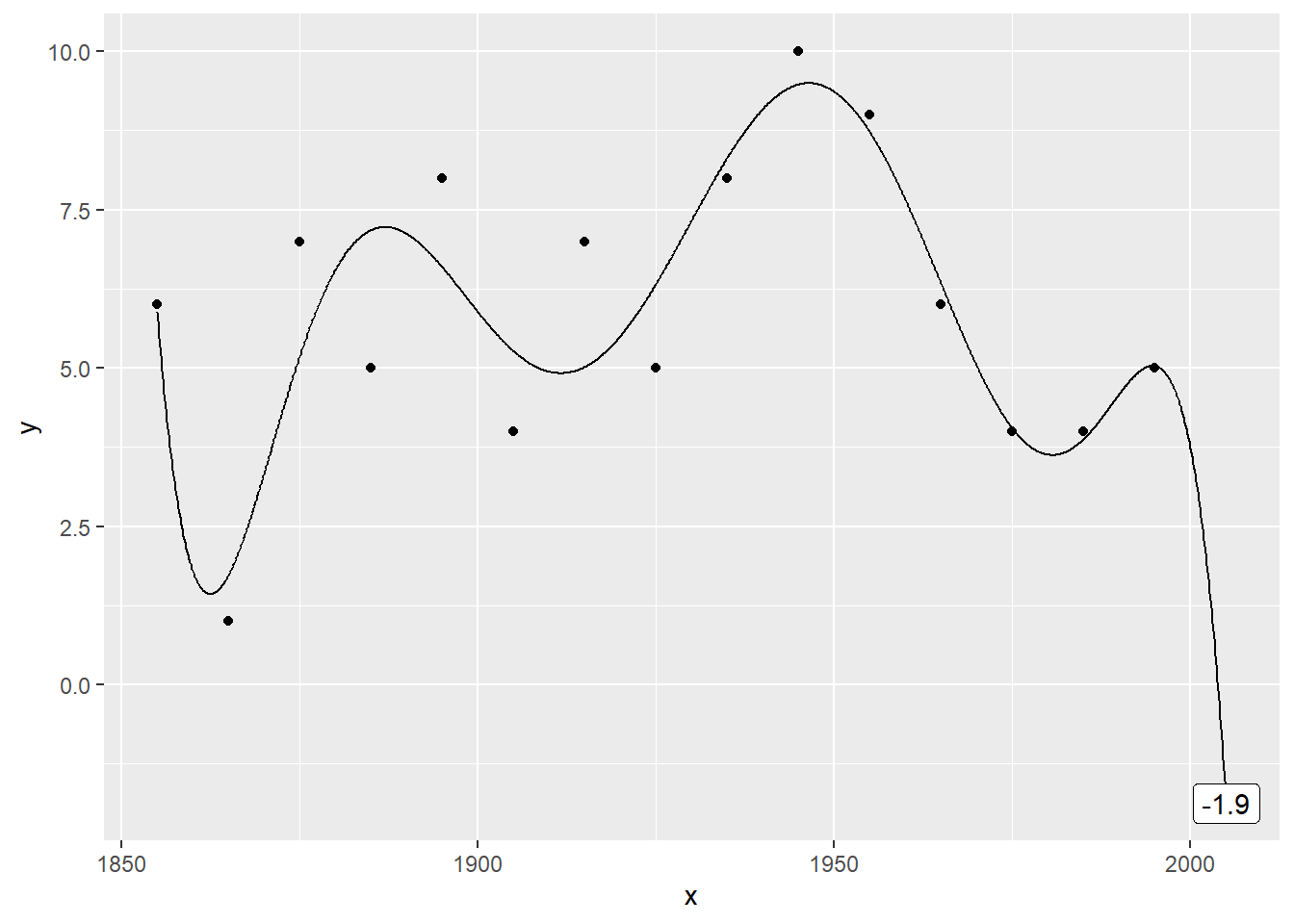The eigth order polynomial trend also produces a negative prediction (-12.8087912).

### Plot the data with a 9th order polynomial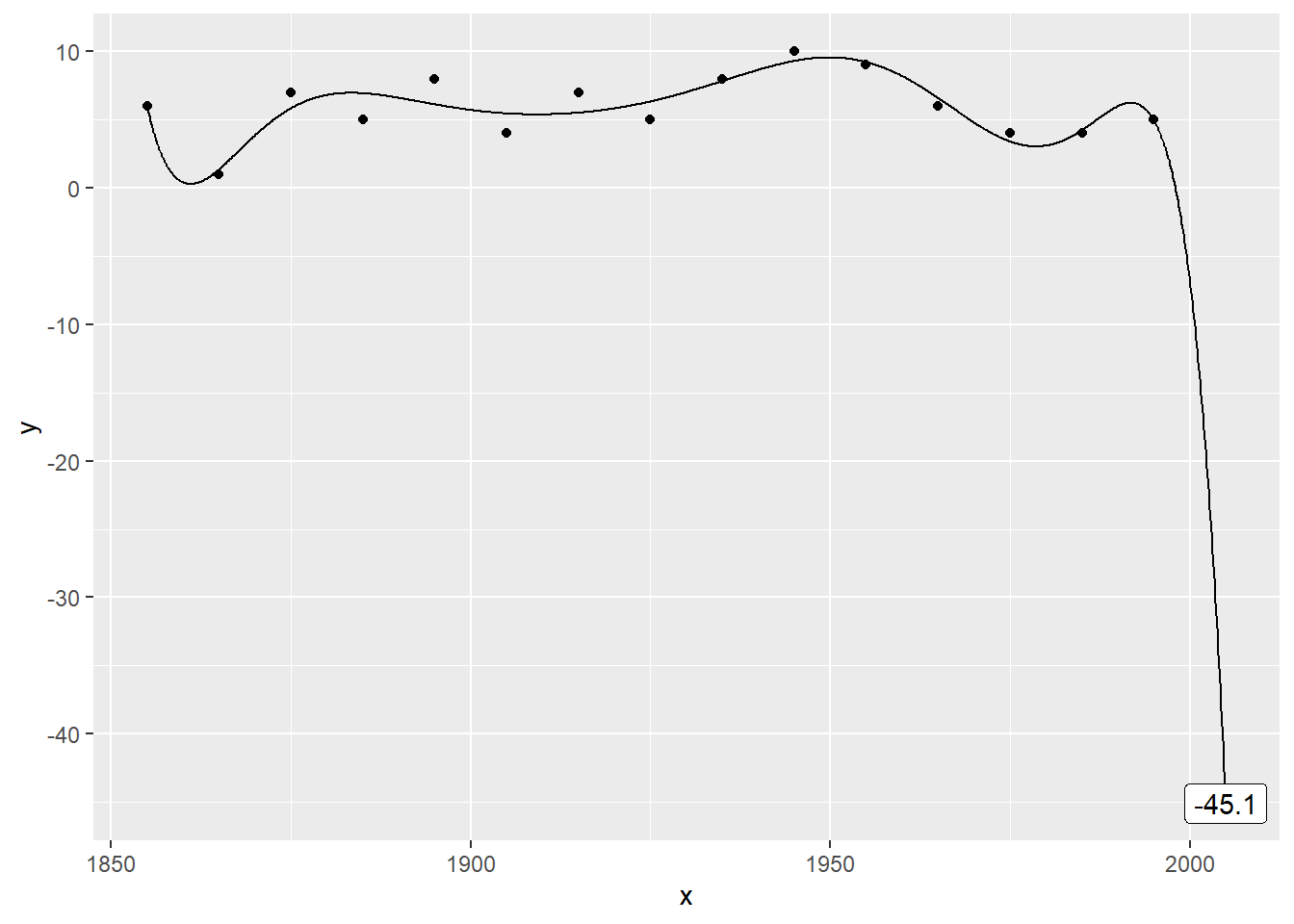Here’s a ninth order polynomial trend. The prediction is so extreme (50.7472527) as to be ridiculous.

### Plot the data with a 10th order polynomial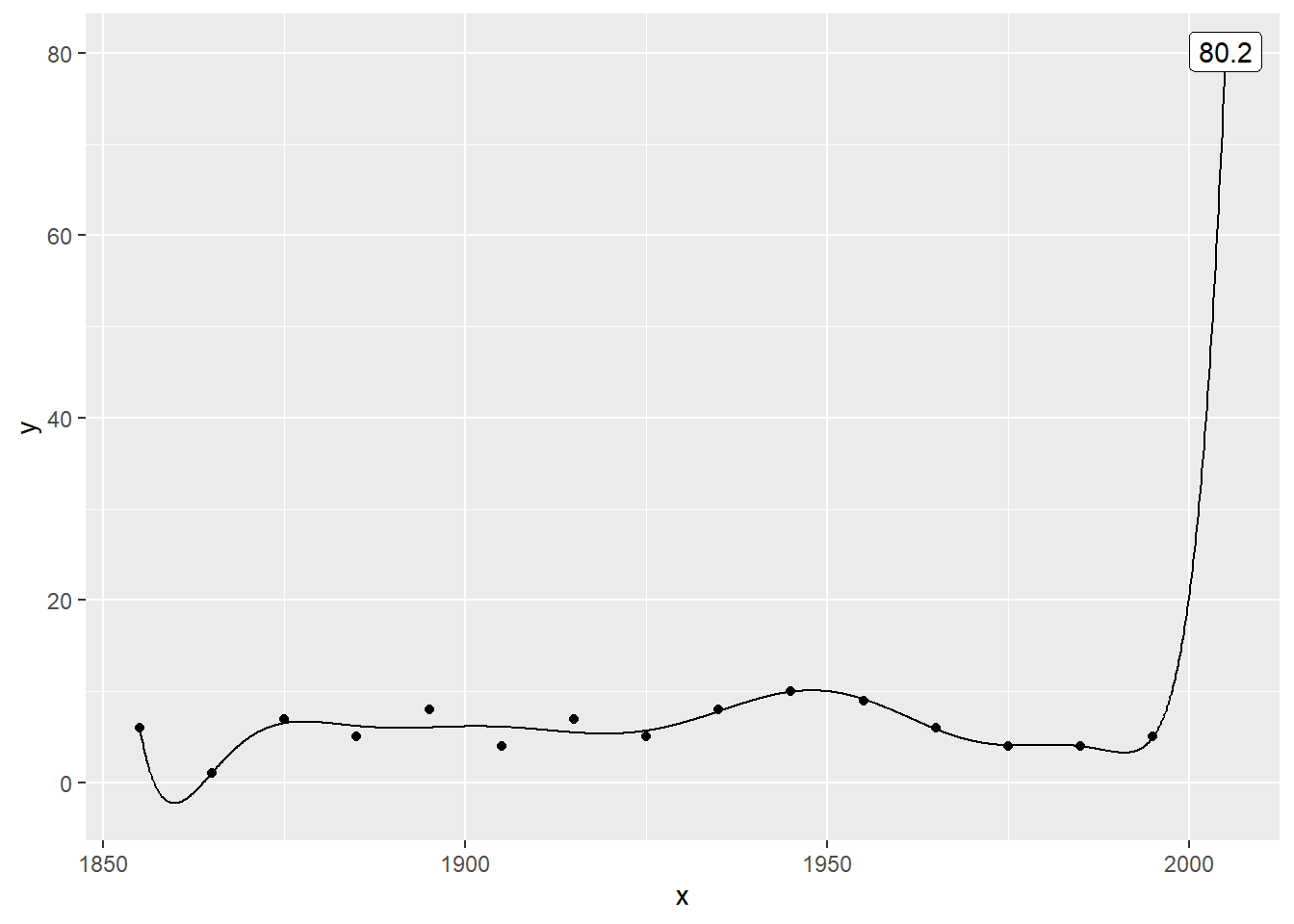For the record, here are the tenth…

### Plot the data with a 11th order polynomial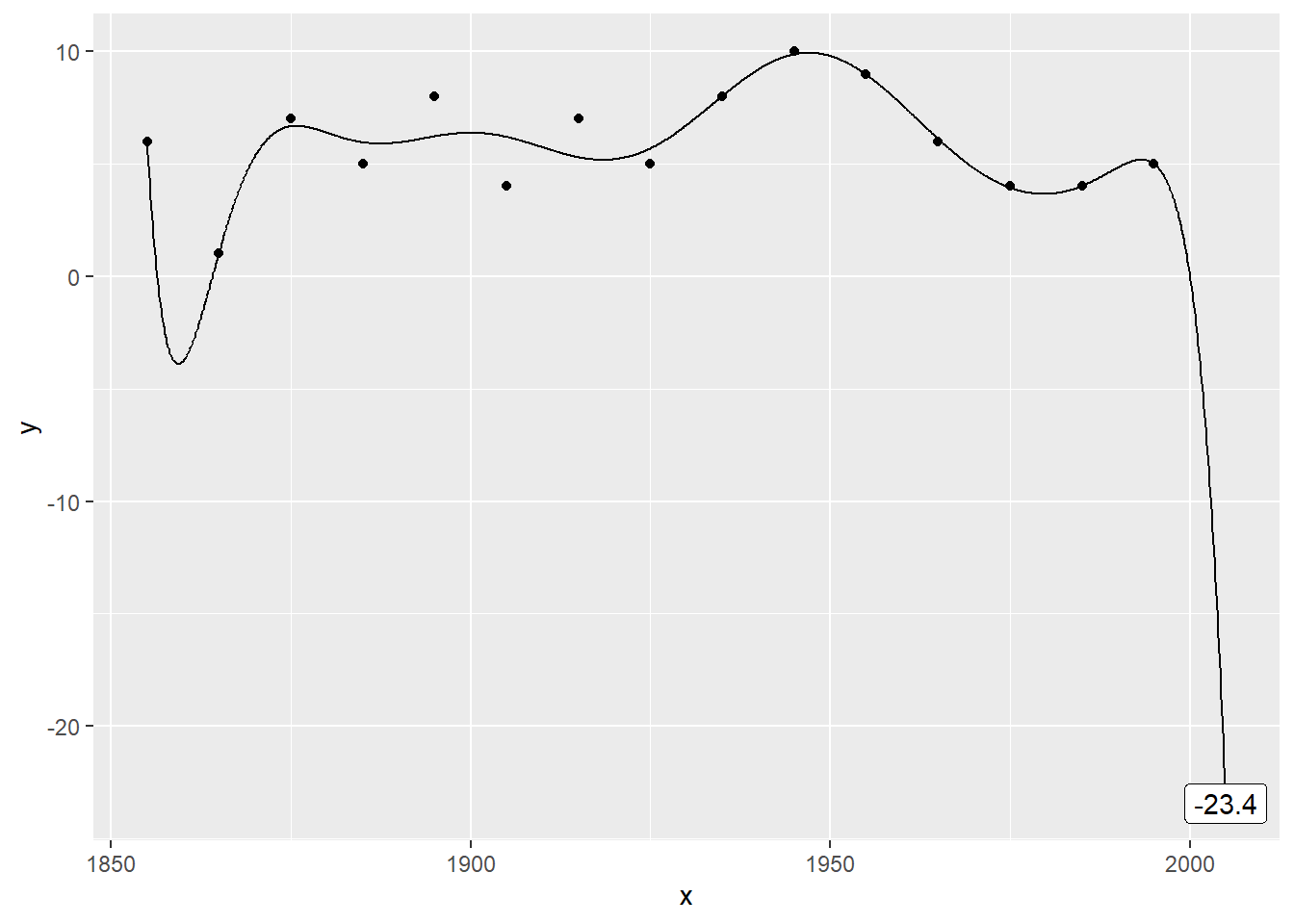… eleventh …

### Plot the data with a 12th order polynomial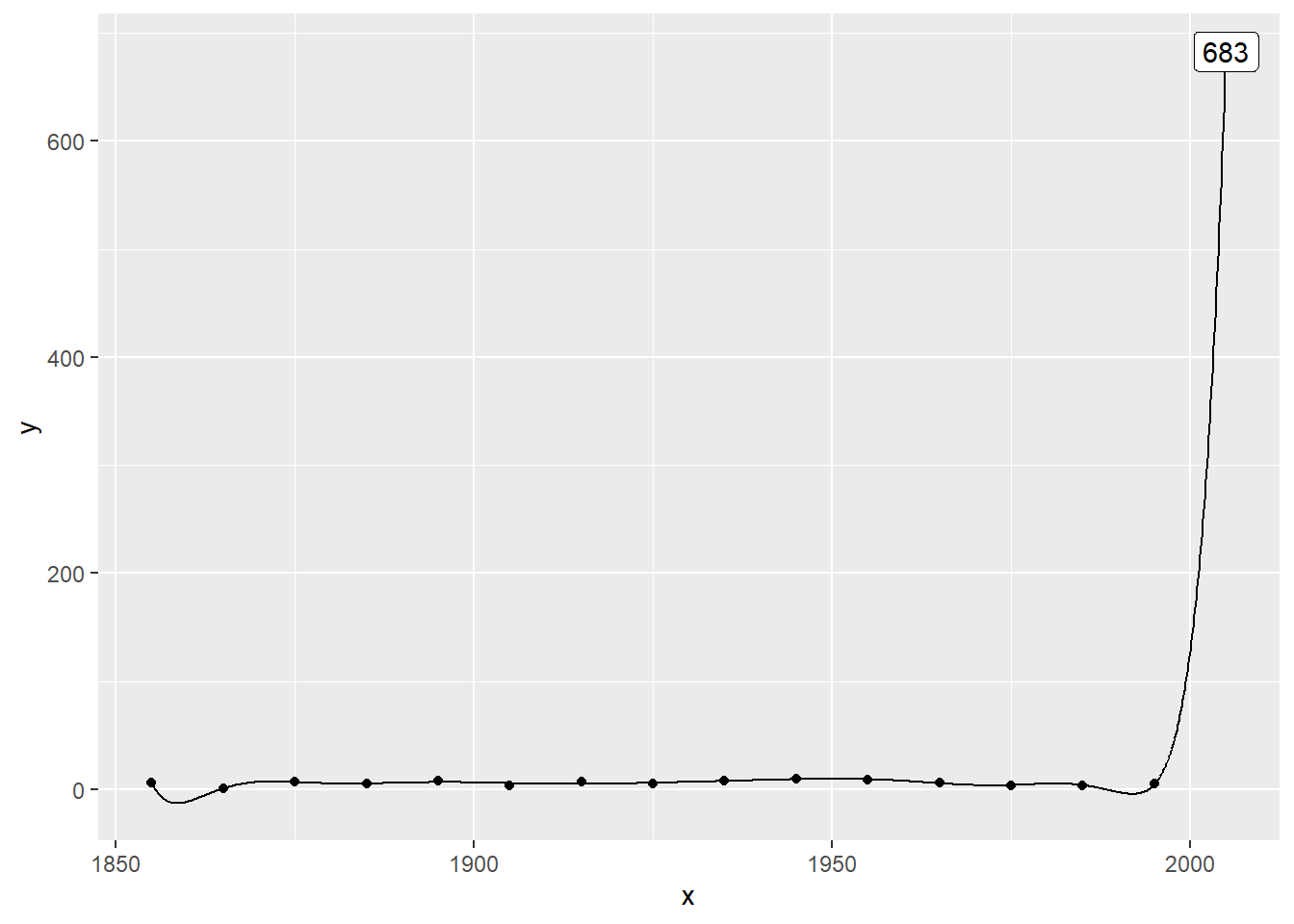… twelfth …

### Plot the data with a 13th order polynomial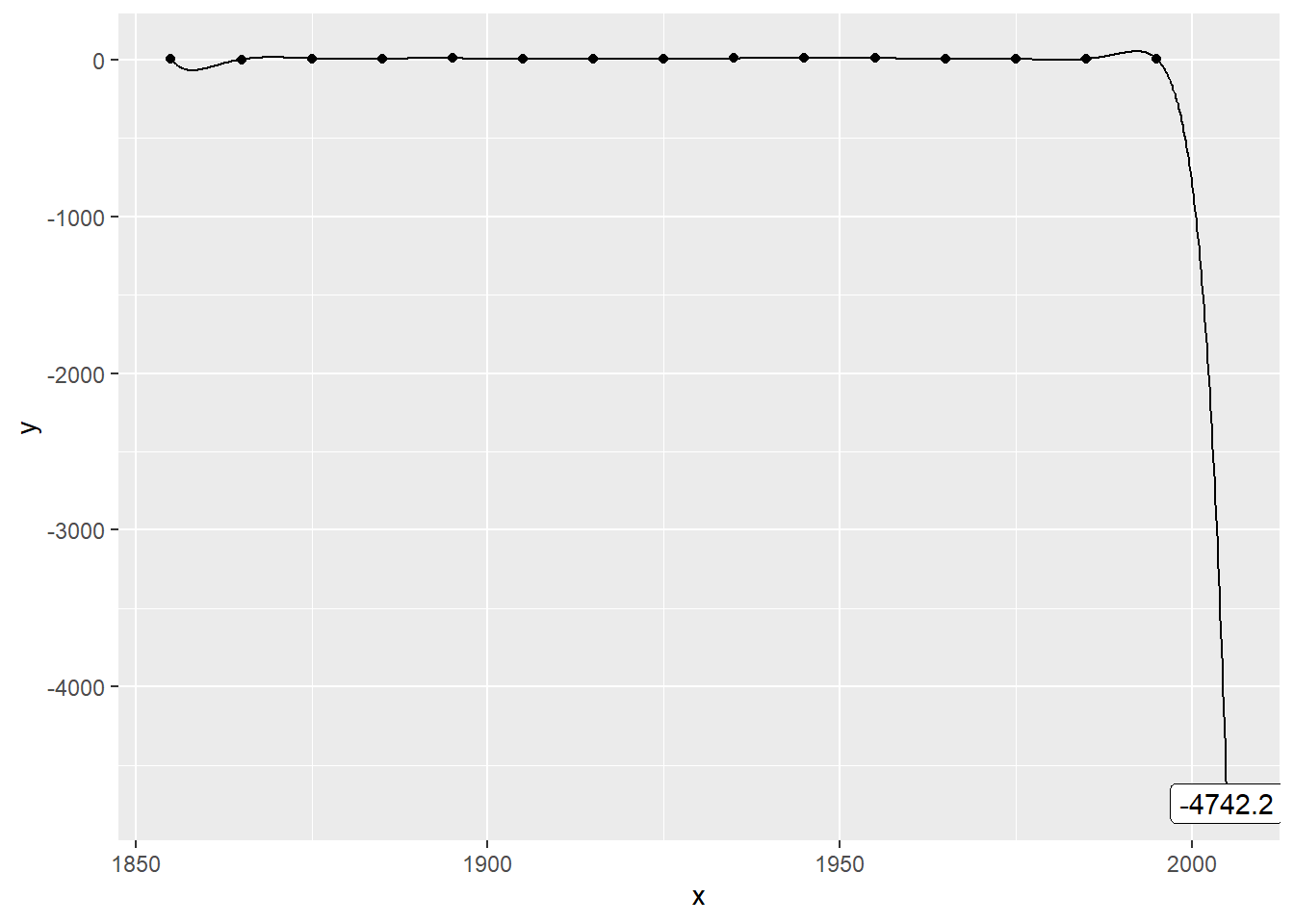… thirteenth …

### Plot the data with a 14th order polynomial… and fourteenth order polynomials.

suppressMessages(suppressWarnings(library(broom)))
suppressMessages(suppressWarnings(library(dplyr)))
suppressMessages(suppressWarnings(library(ggplot2)))
suppressMessages(suppressWarnings(library(knitr)))
suppressMessages(suppressWarnings(library(magrittr)))
knitr::opts_chunk$set(echo=FALSE) Here are the libraries that I needed for this work. ### Code (read and print) fn <- "https://dasl.datadescription.com/download/data/3279" raw_data <- read.delim(file=fn, header=TRUE, sep="\t") raw_data[1:8, ] raw_data[9:16, ] This code reads data directly from the website and prints out the data in two pieces. ### Code (plot data points) df <- data.frame(x=seq(1855, 1995, by=10), y=raw_data$Count[1:15])
ggplot(df, aes(x, y)) +
geom_point() -> point_plot
plot(point_plot)

Here’s the code for plotting the data points. I save the graph in an object so I can add trend lines later on.

### Code that I used

add_trend <- function(point_plot, df, degree) {
x_grid <- data.frame(x=seq(1855, 2005, by=0.1))
lm(y~poly(x, degree), data=df) %>%
augment(newdata=x_grid) -> yhat
yhat_2005 <- filter(yhat, x==2005)
prediction <<- yhat_2005\$.fitted
point_plot +
geom_line(data=yhat, aes(x, .fitted)) +
geom_label(
data=yhat_2005,
aes(x, .fitted, label=round(.fitted, 1)))
}
add_trend(point_plot, df, 1)

Here’s the code for adding a trend line to the graph. I am setting up a flexible function that can print the trend from any polynomial.

### Code that I used

add_trend(point_plot, df, 2)
add_trend(point_plot, df, 3)
add_trend(point_plot, df, 4)
add_trend(point_plot, df, 5)
add_trend(point_plot, df, 6)
add_trend(point_plot, df, 7)
add_trend(point_plot, df, 8)
add_trend(point_plot, df, 9)
add_trend(point_plot, df, 10)
add_trend(point_plot, df, 11)
add_trend(point_plot, df, 12)
add_trend(point_plot, df, 13)
add_trend(point_plot, df, 14)

It took a bit of work to put everything in a function, but now you can produce a quadratic or higher level trend rather than a linear trend with just a single line of code.